Question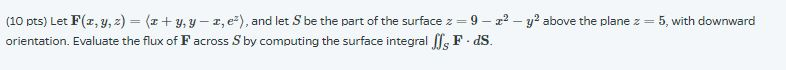​​​​​​​PLEASE SHOW ALL WORK NEATLY! THANK YOU!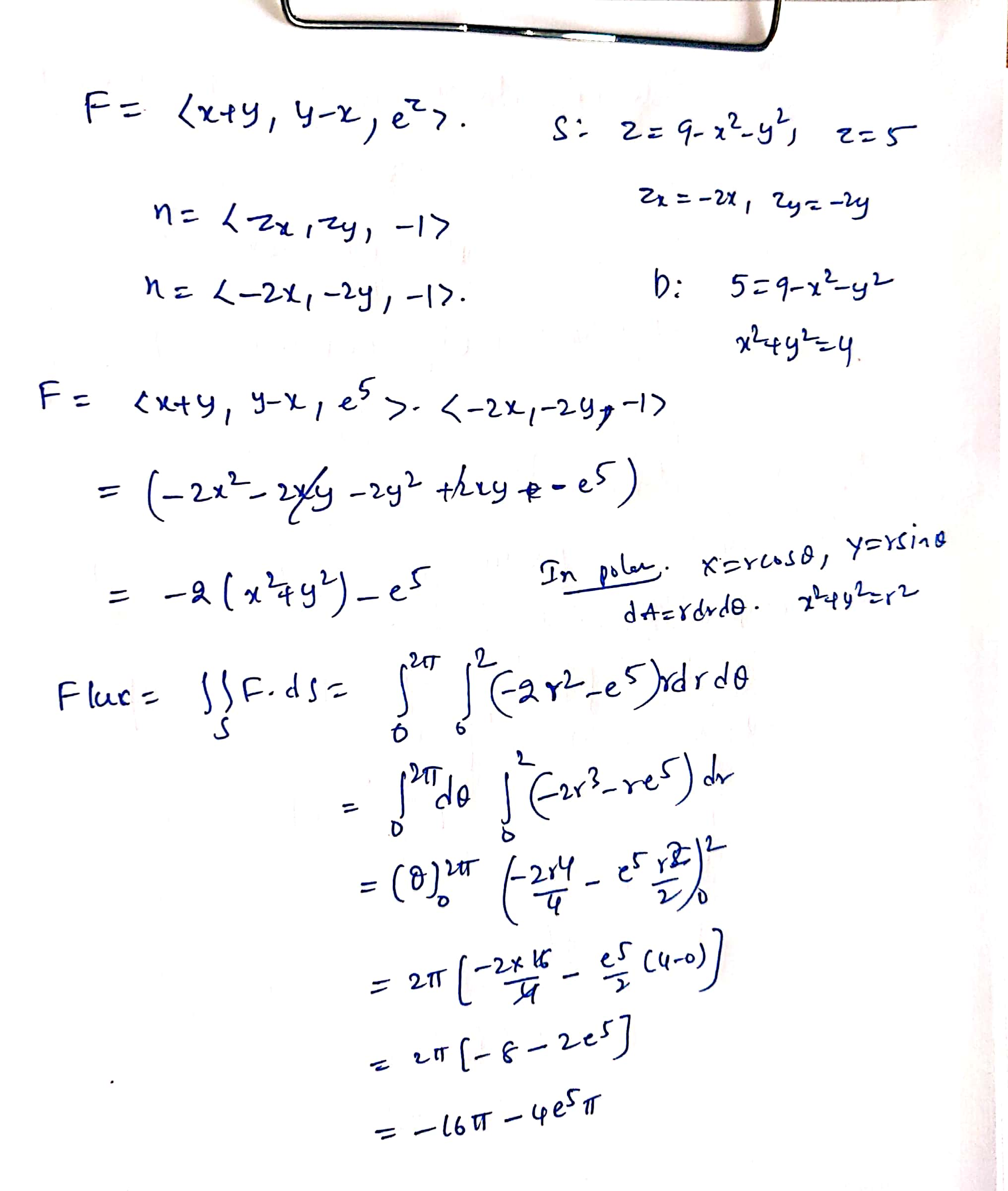#### Earn Coins

Coins can be redeemed for fabulous gifts.

Similar Homework Help Questions
• ### Consider the vector field F(x, y, z) = (z arctan(y2), 22 In(22 +1), 32) Let the...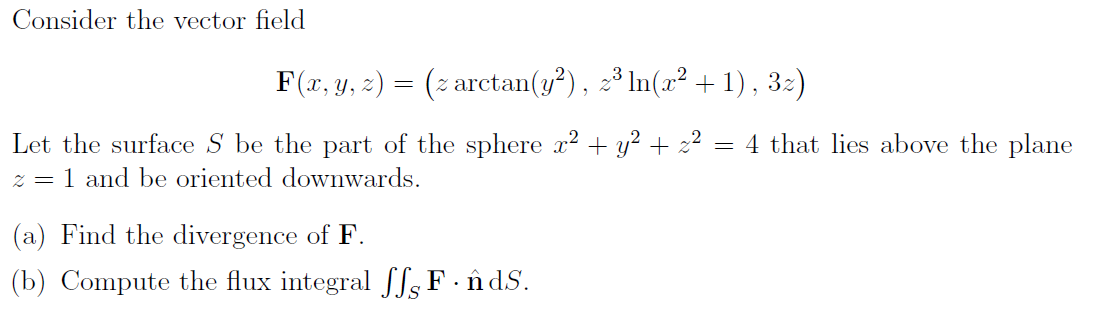Consider the vector field F(x, y, z) = (z arctan(y2), 22 In(22 +1), 32) Let the surface S be the part of the sphere x2 + y2 + x2 = 4 that lies above the plane 2=1 and be oriented downwards. (a) Find the divergence of F. (b) Compute the flux integral SS. F . ñ ds.

• ### please just the final answer for both Evaluate the surface Integral || 5. ds for the...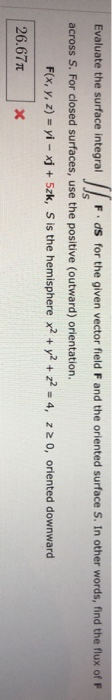please just the final answer for both Evaluate the surface Integral || 5. ds for the given vector fleld F and the oriented surface S. In other words, find the flux of F across S. For closed surfaces, use the positive (outward) orientation. F(x, y, z) = yi - xj + Szk, S is the hemisphere x2 + y2 + y2 = 4, 220, oriented downward 26.677 X Evaluate the surface integral llo F.ds for the given vector field F...

• ### Evaluate the surface integral F dot dS for the given vector field F and the oriented...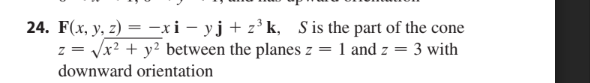Evaluate the surface integral F dot dS for the given vector field F and the oriented surface S. In other words, find the flux of F across S. For closed surfaces, use the positive (outward) orientation. 24. F(x, y, z) = -xi - yj + z’k, S is the part of the cone z = x2 + y2 between the planes z 1 and 2 3 with downward orientation

• ### (23 pts) Let F(x, y, z) = ?x + y, x + y, x2 + y2?,...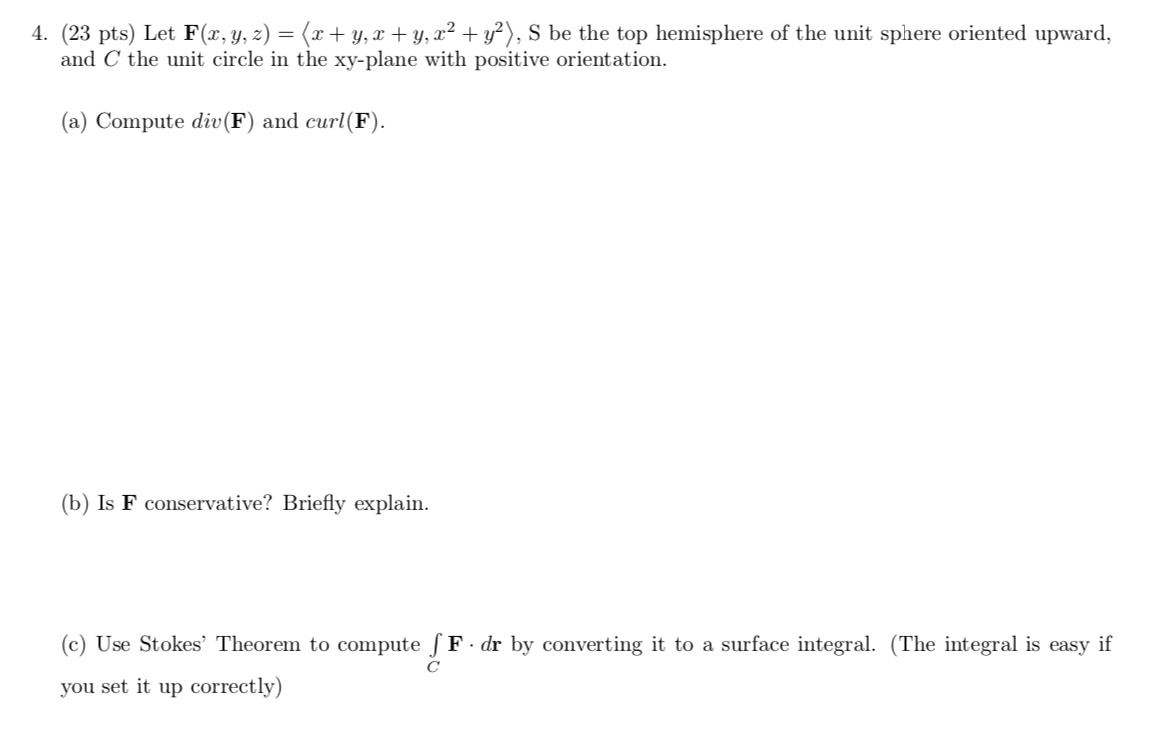(23 pts) Let F(x, y, z) = ?x + y, x + y, x2 + y2?, S be the top hemisphere of the unit sphere oriented upward, and C the unit circle in the xy-plane with positive orientation. (a) Compute div(F) and curl(F). (b) Is F conservative? Briefly explain. (c) Use Stokes’ Theorem to compute ? F · dr by converting it to a surface integral. (The integral is easy if C you set it up correctly) 4. (23 pts)...

• ### 2. Evaluate the surface integral [[Fids. (a) F(x, y, z) - xi + yj + 2zk,...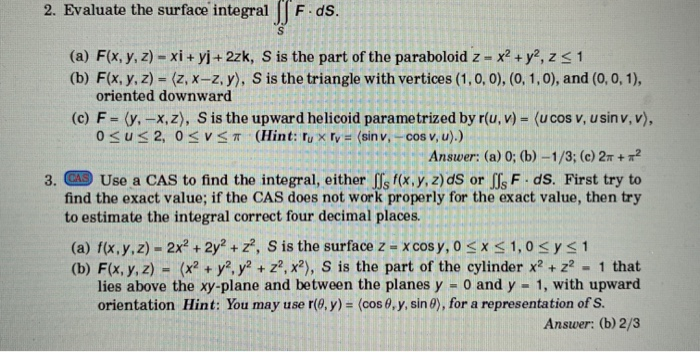2. Evaluate the surface integral [[Fids. (a) F(x, y, z) - xi + yj + 2zk, S is the part of the paraboloid z - x2 + y2, 251 (b) F(x, y, z) = (z, x-z, y), S is the triangle with vertices (1,0,0), (0, 1,0), and (0,0,1), oriented downward (c) F-(y. -x,z), S is the upward helicoid parametrized by r(u, v) = (UCOS v, usin v,V), osus 2, OSVS (Hint: Tu x Ty = (sin v, -cos v, u).)...

• ### [25 pts) Let F(x, y, z) = x?i + xyj + zzk be a vector field...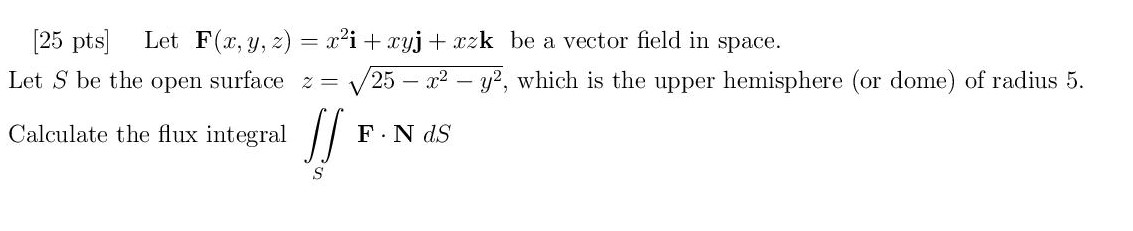[25 pts) Let F(x, y, z) = x?i + xyj + zzk be a vector field in space. Let S be the open surface 2 = 25 -x2 - y2, which is the upper hemisphere (or dome) of radius 5. Calculate the flux integral ] F.N ds S

• ### Questions. Please show all work. 1. Consider the vector field F(x, y, z) (-y, x-z, 3x + z)and the surface S, which is the part of the sphere x2 + y2 + z2 = 25 above the plane z = 3. Let C be the...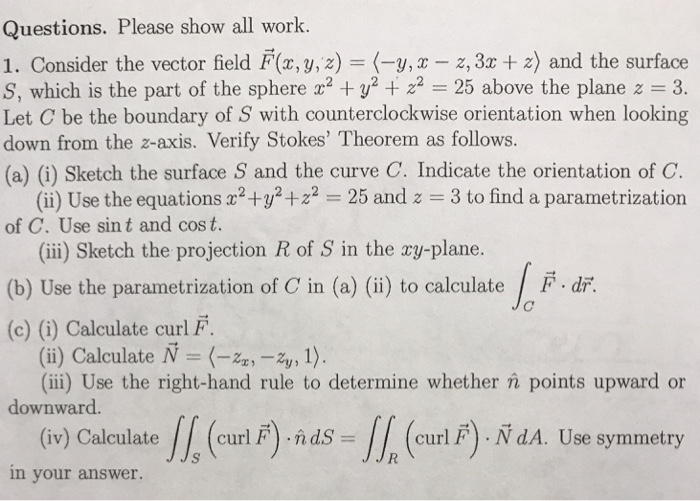Questions. Please show all work. 1. Consider the vector field F(x, y, z) (-y, x-z, 3x + z)and the surface S, which is the part of the sphere x2 + y2 + z2 = 25 above the plane z = 3. Let C be the boundary of S with counterclockwise orientation when looking down from the z-axis. Verify Stokes' Theorem as follows. (a) (i) Sketch the surface S and the curve C. Indicate the orientation of C (ii) Use the...

• ### Evaluate the surface integral    S F · dS for the given vector field F and...

Evaluate the surface integral    S F · dS for the given vector field F and the oriented surface S. In other words, find the flux of F across S. For closed surfaces, use the positive (outward) orientation. F(x, y, z) = x i − z j + y k S is the part of the sphere x2 + y2 + z2 = 36 in the first octant, with orientation toward the origin

• ### Evaluate the surface integral S F · dS for the given vector field F and the...

Evaluate the surface integral S F · dS for the given vector field F and the oriented surface S. In other words, find the flux of F across S. For closed surfaces, use the positive (outward) orientation. F(x, y, z) = xzey i − xzey j + z k S is the part of the plane x + y + z = 7 in the first octant and has upward orientation.

• ### Could you do number 4 please. Thanks 1-8 Evaluate the surface integral s. f(x, y, z) ds Vx2ty2 -vr+) 1. f(x, y, z) Z2; ơ is the portion of the cone z between the planes z 1 and z 2 1 2. f(x, y, z)...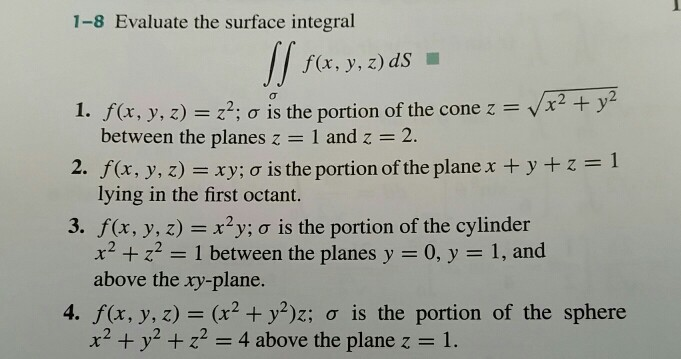Could you do number 4 please. Thanks 1-8 Evaluate the surface integral s. f(x, y, z) ds Vx2ty2 -vr+) 1. f(x, y, z) Z2; ơ is the portion of the cone z between the planes z 1 and z 2 1 2. f(x, y, z) xy; ơ is the portion of the plane x + y + z lying in the first octant. 3. f(x, y, z) x2y; a is the portion of the cylinder x2z2 1 between the planes...